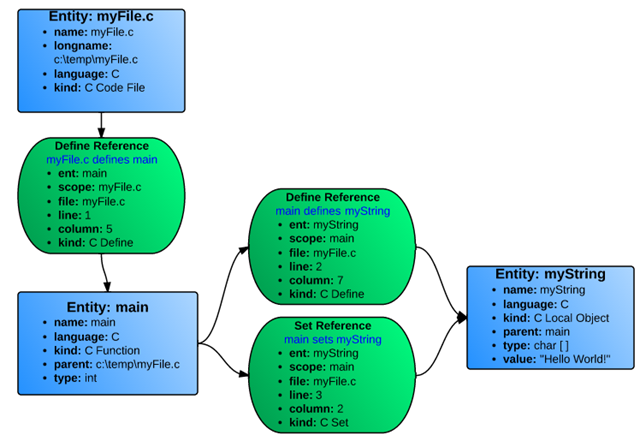Entity vs. Reference

Now that we know what an API file looks like and how to open an Understand project, we can look at the actual data from that project. Most of the data captured by Understand involves Entities and References.

Entity: An Entity is anything in the code that Understand captures information on: i.e. A file, a class, a variable, a function, etc.In the Perl API Entities are represented with the Understand::Ent class. In Python it is the Understand.Ent class.

Reference: A specific place where an entity appears in the code. A reference is always defined as a relationship between two entities. e.g. function Bar is called on line 14 of function Foo.In the Perl API References are represented with the Understand::Ref class.

In Python it is the Understand.Ref class.

Every entity and reference has a unique set of attributes that can be queried by the API. A few of the attributes you can view for an entity would be its name, its type, any associated comments, what kind of entity it is and if it has them: its parent entity and its parameters.

On the other hand, a reference would have both of the entities associated with it as well as the file, line, and column where the reference occurs and what kind of reference it is.

To help visualize this, let’s use this simple C code:

myFile.c

```void main(){
char myString[];
myString = “Hello World!”;
}```

Understand would identify three entities (blue) and three references (green):Since all references are relationships between two objects, the references are actually stored going both directions. So each reference kind has an opposite: Define and DefineIn, Set and SetBy, Call and Callby, etc. Here are the same entities with their reverse references.To get a list of all of the entities in the project, use the command \$db->ents() or db.ents() for python. To get a list of all of the references for an entity, \$ent->refs() or ent.refs().

Usually though, we don’t want all of the entities or references, just some of them, and that is where kind filters come in.

Kind Filters

A Kind Filter is simply a string used to filter a list in Understand. We’ve already seen a small example in the first script we wrote:

`\$db->ents(“file”));`

As noted earlier, the \$db–>ents() command by itself returns a list of all of the entities in Understand, from loop variables to namespaces and everything in between. Specifying the “file” kind string creates a filter, and everything that doesn’t match the filter is rejected. So only files are returned in this case.

You can add simple logic to the string as well:

tilde (~) means NOT

a space means AND

comma(,) can act as a top level OR

So, in our example if we wanted all classes and functions listed instead of files, we would just change the filter:

`\$db->ents(“class, function”));`

Which reads as: return any entity that is a class OR that is a function.

An unresolved entity is one that Understand has the declaration for, but not the definition (printf is a common example). This filter would return all non-volatile public functions that are defined in the project.

`\$db->ents(“function public ~unresolved ~volatile”));`

An exhaustive list of kinds for each language is included at the end of the perl API documentation here. Alternatively, if you have a sample entity in mind and you want to know its full kind string, you can use this plugin in the GUI to query it.

An Example

List the file and line where each function in the project is defined. This example assumes the Understand database has been opened already (see the templates at the end of the first tutorial).

First get a list of all functions defined in the project:

`my @ents = \$db->ents(“function ~unresolved ~unknown”);`

Then sort the list and iterate through it:

`foreach my \$ent (sort {lc(\$a->longname()) cmp lc(\$b->longname());} @ents){`

Next, get the reference where each function is defined. If it doesn’t have a definition, skip to the next function:

```my \$ref = \$ent->ref(“definein”);
next unless \$ref;```

Next, print the name of the function and it’s parameters:

`print \$ent->longname. “(”. \$ent->parameters().")\n";`

And finally print the file and line number where the reference occurs:

`print " “.\$ref->file->relname.”(".\$ref->line.")\n";`

So the full thing in Perl:

```my @ents = \$db->ents("function ~unknown ~unresolved");
foreach my \$ent (sort {lc(\$a->longname()) cmp lc(\$b->longname());} @ents){
my \$ref = \$ent->ref("definein");
next unless \$ref;
print \$ent->longname. "(". \$ent->parameters().")\n";
print " “.\$ref->file->relname.”(".\$ref->line.")\n";
}```

And in Python:

```ents = db.ents("function ~unknown ~unresolved")
for ent in sorted(ents,key= lambda ent: ent.longname()):
ref = ent.ref(“definein”);
if ref is None:
continue;
print (ent.longname(),"(",ent.parameters(),")");
print (" ",ref.file().relname(),"(",ref.line(),")");```

Next up, part 3: Lexers and Lexemes